# AP Physics C: Mechanics : Calculating Motion in Two Dimensions

## Example Questions

### Example Question #1 : Calculating Motion In Two Dimensions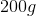ball is thrown horizontally from the top of a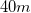high building. It has an initial velocity of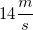and lands on the groundaway from the base of the building. Assuming air resistance is negligible, which of the following changes would cause the range of this projectile to increase?

I. Increasing the initial horizontal velocity

II. Decreasing the mass of the ball

III. Throwing the ball from an identical building on the moon

Possible Answers:

I and II

I only

II only

I, II, and III

I and III

Correct answer:

I and III

Explanation:

Relevant equations: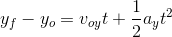Choice I is true because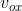is proportional to the range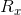, so increasingincreasesifis constant. This relationship is given by the equation: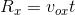Choice II is false because the motion of a projectile is independent of mass.

Choice III is true because the vertical acceleration on the moon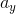would be less. Decreasingincreases the time the ball is in the air, thereby increasingifis constant. This relationship is also shown in the equation:### Example Question #2 : Calculating Motion In Two Dimensions

Water emerges horizontally from a hole in a tank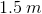above the ground. If the water hits the ground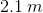from the base of the tank, at what speed is the water emerging from the hole? (Hint: Treat the water droplets as projectiles.)

Possible Answers: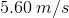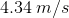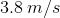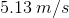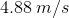Correct answer:Explanation:

To understand this problem, we have to understand that the water has a x-velocity and a y-veloctiy. The x-velocity never changes.

First we want to find the time it took for the water to hit the ground. We can use this equation: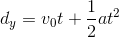We know that the y-velocity is 0 to start with, acceleration is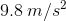and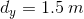.

Substituting into the equation, we get: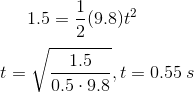Next we have to substitute the time into the equation for the x component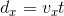We know that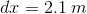and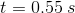, so we can conclude that: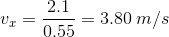### All AP Physics C: Mechanics Resources